Courses

# Test: Permutation, Combination & Probability - 1 (2019-2017)

## 10 Questions MCQ Test UPSC Topic Wise Previous Year Questions | Test: Permutation, Combination & Probability - 1 (2019-2017)

Description
This mock test of Test: Permutation, Combination & Probability - 1 (2019-2017) for UPSC helps you for every UPSC entrance exam. This contains 10 Multiple Choice Questions for UPSC Test: Permutation, Combination & Probability - 1 (2019-2017) (mcq) to study with solutions a complete question bank. The solved questions answers in this Test: Permutation, Combination & Probability - 1 (2019-2017) quiz give you a good mix of easy questions and tough questions. UPSC students definitely take this Test: Permutation, Combination & Probability - 1 (2019-2017) exercise for a better result in the exam. You can find other Test: Permutation, Combination & Probability - 1 (2019-2017) extra questions, long questions & short questions for UPSC on EduRev as well by searching above.
QUESTION: 1

### The number of parallelograms that can be formed from a set of four parallel lines intersecting another set of four parallel lines, is 

Solution:

Number of parallelograms that can be formed from 'm' parallel horizental and 'n' parallel vertical lines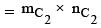Here m = n = 4.
So, Number of prallelograms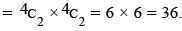QUESTION: 2

### Suppose you have sufficient amount of rupee currency in three denominations: ₹1, ₹10 and ₹ 50. In how many different ways can you pay a bill of ₹ 107? 

Solution:

Number of Denominations are shown in the table: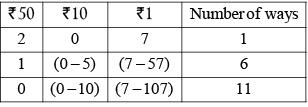Total number of ways = 1 + 6 + 11 = 18.

QUESTION: 3

### A printer numbers the pages of a book starting with 1 and uses 3089 digits in all. How many pages does the book have? 

Solution:

Pages till 9 uses 9 digits i.e.; 1 to 9.
Pages from 10 to 99 uses 2 digits each.
Number of pages = (99 – 10) + 1 = 90
Number of digits = 2 × 90 = 180.
Pages from 100 to 999 uses 3 digits each.
Number of pages = 999 – 100 + 1 = 900.
Number of digits = 3 × 900 = 2700.
Pages from 1000 to 9999 uses 4 digits each.
Remaining digits = 3089 – 2700 – 180 – 9 = 200.
Remaining number of pages = (200/4) = 50.
Hence, total number of pages = 999 + 50 = 1049.

QUESTION: 4

How many triplets (x, y, z) satisfy the equation x + y + z = 6, where x, y and z are natural numbers?



Solution: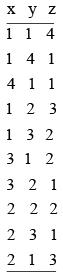Total Number of triplets = 10.

QUESTION: 5

Each face of a cube can be painted in black or white colours. In how many different ways can the cube be painted?



Solution:

A cube has 6 symmetric faces.
Number of faces of a cube coloured by: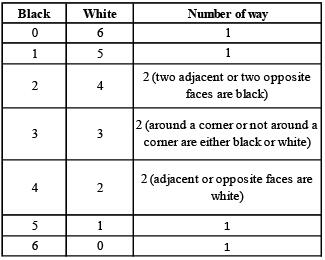Hence, total number of ways
= 1 + 1 + 2 + 2 + 2 + 1 + 1  = 10

QUESTION: 6

A bag contains 15 red balls and 20 black balls. Each ball is numbered either 1or 2 or 3. 20% of the red balls are numbered 1 and 40% of them are numbered 3. Similarly, among the black balls, 45% are numbered 2 and 30% are numbered 3. A boy picks a ball at random. He wins if the ball is red and numbered 3 or if it is black and numbered 1 or 2. What are the chances of his winning?



Solution:

Total number of balls 15 + 20 = 35
Total number of red balls numbered 3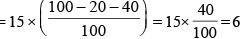Total number of black ball numbered 1 or 2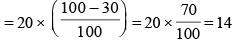Required chances =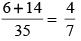QUESTION: 7

While writing all the numbers from 700 to 1000, how many numbers occur in which the digit at hundred’s place is greater than the digit at ten’s place, and the digit at ten’s place is greater than the digit at unit’s place?



Solution: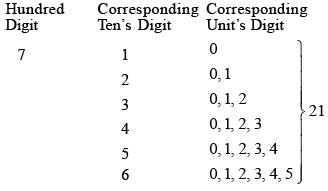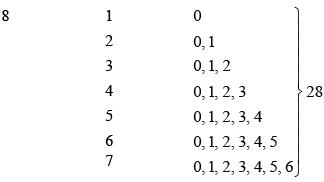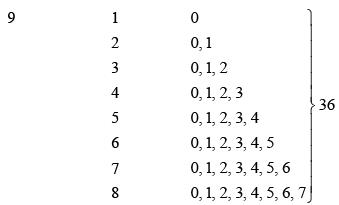So, total numbers = 21 + 28 + 36 = 85

QUESTION: 8

How many diagonals can be drawn by joining the vertices of an octagon?



Solution:

Number of diagonals in an octagon = 8C2 - 8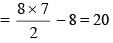QUESTION: 9

For a sports meet, a winners’ stand comprising three wooden blocks is in the following form :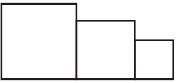There are six different colours available to choose from and each of the three wooden blocks is to be painted such that no two of them has the same colour. In how many different ways can the winners’ stand be painted?



Solution:

Required number of ways = 6!/3! = 120

QUESTION: 10

If 2 boys and 2 girls are to be arranged in a row so that the girls are not next to each other, how many possible arrangements are there?



Solution:

—B1 —B2
2 boys can take their seats in 2! ways and 3 girls can take the remaining 3 seats in 3C2 x 2! ways.
Hence, 2! x 3C2 x 2! = 12 ways.